# transversal

## Snevily's conjecture ★★★

Author(s): Snevily

Conjecture   Letbe an abelian group of odd order and let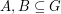satisfy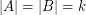. Then the elements of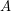and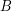may be orderedand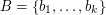so that the sums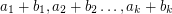are pairwise distinct.

Keywords: addition table; latin square; transversal

## Rota's basis conjecture ★★★

Author(s): Rota

Conjecture   Letbe a vector space of dimensionand letbe bases. Then there existdisjoint transversals ofeach of which is a base.

Keywords: base; latin square; linear algebra; matroid; transversal